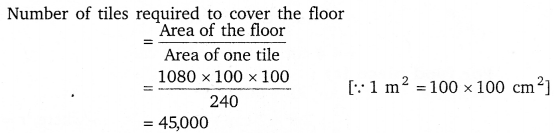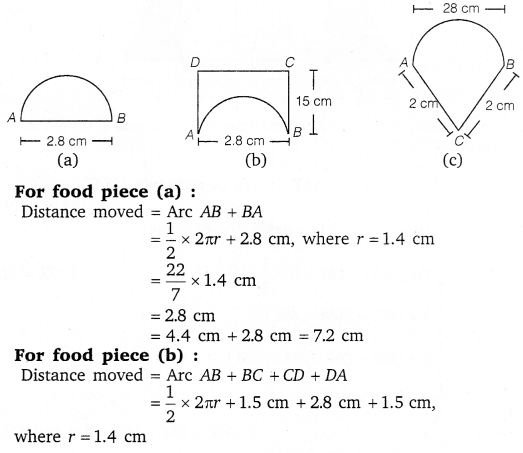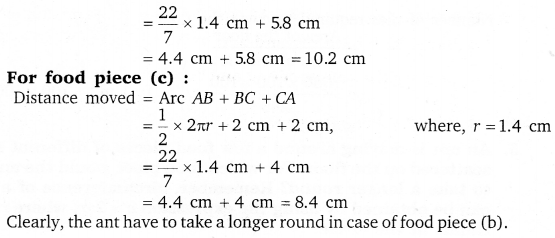# Class 8 Maths NCERT Solutions for Chapter – 11 Mensuration Ex – 11.1

## Mensuration

Question 1.
A square and a rectangular field with measurements as given in the following figure have the same perimeter. Which field has a larger area?

Solution:

Let x be the breadth of the rectangle. It is given that the perimeter of a rectangle = perimeter of the square.
∴ 2 (80 + x) = 4 x 60
⇒ 80 + x = 120
⇒ x = 120 – 80 =40
i.e., breadth of the rectangle = 40 m
Now area of the square = (60 x 60) m = 3600 m2
and the area of the rectangle = (40 x 80) m2 = 3200 m2
Hence, the square field has a larger area.

Question 2.
Mrs. Kaushik has a square plot with the measurement as shown in the figure. She wants to construct a house in the middle of the plot. A garden is developed around the house. Find the total cost of developing a garden around the house at the rate of ₹ 55 per m2.

Solution:

Area of the garden = Area of the outer square – Area of the inner rectangle
= 25 x 25 m2 – 20 x 15 m2
= (625 – 300) m2 = 325 m2
Cost of developing a garden at the rate of ₹ 55 per sq. metre
= ₹ (55 x 325) = ₹ 17,875

Question 3.
The shape of a garden is rectangular in the middle and semi circular at the ends as shown in the diagram. Find the area and the perimeter of this garden [Length of rectangle is 20 – (3.5 + 3.5) metres].

Solution:

Total area of the garden = Area of the rectangular portion + The sum of the areas of the pair of semi-circlesQuestion 4.
A flooring tile has the shape of a parallelogram whose base is 24 cm and the corresponding height is 10 cm. How many such tiles are required to cover a floor of area 1080 m2?

Solution:
Area of one tile = base x height
= (24 x 10) cm2 = 240 cm2Question 5.
An ant is moving around a few food pieces of different shapes scattered on the floor. For which food piece would the ant have to take a longer round? Remember, circumference of a circle can be obtained by using the expression c = 2πr, where r is the radius of the circle.

Solution:

A, B, C and D in the given figures as shown. Let A be the point in each figure from where the ant start moving on the food pieces. She is to reach the initial point after moving around the boundary of each food piece.